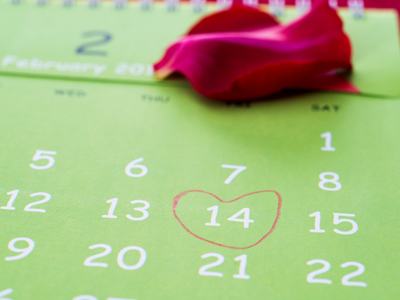7 x 2 = 14.

# The 02 Times Table

You probably think that the two times table is too easy but learning it is vitally important. You may believe you will never need to use the two times table in life - but think again! It's one of the most simple parts of maths and that applies also to KS3 Maths.

The two times' table is one of the easiest to learn as multiplying by 2 is the same as doubling a number. The time taken for something to double, the doubling time, is an important piece of information in a lot of practical situations, e.g. working out inflation, population growth and compound interest.

Don't be too confident when you play this quiz. Yes, the two times table is one of the most basic, but if you take it for granted then you may misread a question and get the answer wrong. Take your time and read each question carefully. You wouldn't want to fail such an easy test now would you?

1.
What is 7 x 2
12
18
15
14
There are 14 days in two weeks - a fortnight
2.
What is 2 x 2
6
8
4
2
When we multiply a number by itself we square it. 2 squared is 4
3.
What is 5 x 2
8
12
10
20
To multiply a number by 5, first times it by 10 and then halve the answer
4.
What is 10 x 2
20
22
16
18
To multiply a number by 10, just put a zero on the end of it
5.
What is 8 x 2
16
12
28
18
16 is also 4 squared
6.
What is 3 x 2
6
10
12
8
6 is also known as half a dozen
7.
What is 6 x 2
8
14
12
10
Another name for 12 is a dozen
8.
What is 9 x 2
14
10
16
18
One way to work this out is to multiply 2 by 10, which is 20, and then subtract 2. This gives the answer 18
9.
What is 1 x 2
2
5
4
3
Any number multiplied by one remains the same
10.
What is 4 x 2
8
14
6
16
Remember - to multiply a number by 2 just double it